The Australian Journal of Mathematical Analysis and Applications

 Home News Editors Volumes RGMIA Subscriptions Authors Contact

ISSN 1449-5910

You searched for fan
Total of 169 results found in site

19: Paper Source PDF document

Paper's Title:

Generalised Models for Torsional Spine Reconnection

Author(s):

Ali Khalaf Hussain Al-Hachami

Department of Mathematics,
College of Education For Pure Sciences,
Wasit University,
Iraq.
E-mail: alhachamia@uowasit.edu.iq

Abstract:

Three-dimensional (3D) null points are available in wealth in the solar corona, and the equivalent is probably going to be valid in other astrophysical situations. On-going outcomes from sun oriented perceptions and from reproductions propose that reconnection at such 3D nulls may assume a significant job in the coronal dynamics. The properties of the torsional spine method of magnetic reconnection at 3D nulls are researched. Kinematic model are created, which incorporate the term ηJ that is spatially localised around the null, stretching out along the spine of the null. The null point is to research the impact of shifting the level of asymmetry of the null point magnetic field on the subsequent reconnection process where past examinations constantly considered a non-nonexclusive radially symmetric null. Specifically we analyse the rate of reconnection of magnetic flux at the spine of null point. Logical arrangements are determined for the enduring kinematic equation, and contrasted and the after effects of torsional spine reconnection models when the current is restricted in which the Maxwell conditions are illuminated. The geometry of the current layers inside which torsional spine reconnection happen is autonomous on the symmetry of the magnetic field. Torsional spine reconnection happens in a thin cylinder around the spine, with circular cross-segment when the fan eigenvalues are extraordinary. The short axis of the circle being along the solid field bearing. Just as it was discovered that the fundamental structure of the method of attractive reconnection considered is unaffected by changing the magnetic field symmetry, that is, the plasma flow is discovered rotational around the spine of null point. The spatiotemporal pinnacle current, and the pinnacle reconnection rate achieved, are found not to rely upon the level of asymmetry.

11: Paper Source PDF document

Paper's Title:

A Simple New Proof of Fan-Taussky-Todd Inequalities

Author(s):

Zhi-Hua Zhang and Zhen-Gang Xiao

Zixing Educational Research Section,
Chenzhou City, Hunan 423400, P. R. China.
Zhi-hua Zhang
Url: http://www.hnzxslzx.com/zzhweb/

Department Of Mathematics, Hunan Institute Of Science And Technology,
Yueyang City, Hunan 423400, P. R. China.
Zhen-gang Xiao

Abstract:

In this paper we present simple new proofs of the inequalities: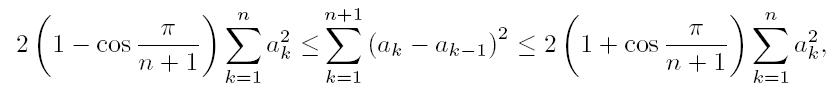which holds for all real numbers a0 = 0, a1, · · · , an, an+1 = 0 and the coefficients 2(1 - cos(π/(n + 1))) and 2(1 + cos(π/(n + 1))) are the best possible; and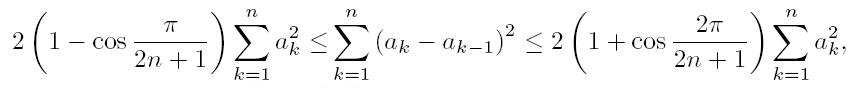which holds for all real numbers a0 = 0, a1, · · · , an and the coefficients 2(1-cos(π/(2n + 1))) and 2(1 + cos(π/(2n + 1))) are the best possible.

11: Paper Source PDF document

Paper's Title:

Composite Variational-Like Inequalities Given By Weakly Relaxed ζ-Semi-Pseudomonotone Multi-Valued Mapping

Author(s):

Syed Shakaib Irfan, Iqbal Ahmad, Zubair Khan and Preeti Shukla

College of Engineering, Qassim University
Buraidah, Al-Qassim,
Saudi Arabia.
E-mail: shakaib@qec.edu.sa

College of Engineering, Qassim University
Buraidah, Al-Qassim,
Saudi Arabia.
E-mail: iqbal@qec.edu.sa

Department of Mathematics,
Integral University Lucknow,
India.
E-mail: zkhan@iul.ac.in

Department of Mathematics,
Integral University Lucknow,
India.
E-mail: shuklapreeti1991@gmail.com

Abstract:

In this article, we introduce a composite variational-like inequalities with weakly relaxed ζ-pseudomonotone multi-valued maping in reflexive Banach spaces. We obtain existence of solutions to the composite variational-like inequalities with weakly relaxed ζ-pseudomon -otone multi-valued maps in reflexive Banach spaces by using KKM theorem. We have also checked the solvability of the composite variational-like inequalities with weakly relaxed ζ-semi-pseudomonotone multi-valued maps in arbitrary Banach spaces using Kakutani-Fan-Glicksberg fixed point theorem.

7: Paper Source PDF document

Paper's Title:

On Zeros of Diagonally Quasiconvex Multifunctions

Author(s):

Zoran D. Mitrović

Faculty of Electrical Engineering,
University of Banja Luka,
78000 Banja Luka, Patre 5
Bosnia and Herzegovina
zmitrovic@etfbl.net

Abstract:

In this paper, we extended the notion of diagonally quasiconvexity for multifunctions and established several existence results for zeros of diagonally quasiconvex multifunctions. As applications we obtain the results of fixed points, coincidence points and best approximations for multifunctions. Using our result we also prove the existence of solutions to the variational-like inequality problem and generalized vector equilibrium problem. The results of this paper generalize some known results in the literature.

7: Paper Source PDF document

Paper's Title:

On Stan Ulam and his Mathematics

Author(s):

Krzysztof Ciesielski and Themistocles M. Rassias

Mathematics Institute, Jagiellonian University,
Ł
jasiewicza 6, 30-348 Kraków,
Poland
Department of Mathematics. National Technical University of Athens,
Zografou Campus, 15780 Athens,
Greece

Krzysztof.Ciesielski@im.uj.edu.pl
trassias@math.ntua.gr

Abstract:

In this note we give a glimpse of the curriculum vitae of Stan Ulam, his personality and some of the mathematics he was involved in.

7: Paper Source PDF document

Paper's Title:

Inequalities for Functions of Selfadjoint Operators on Hilbert Spaces:
a Survey of Recent Results

Author(s):

Sever S. Dragomir1,2

1Mathematics, College of Engineering & Science
Victoria University, PO Box 14428
Melbourne City, MC 8001,
Australia
E-mail: sever.dragomir@vu.edu.au

2DST-NRF Centre of Excellence in the Mathematical and Statistical Sciences,
School of Computer Science & Applied Mathematics,
University of the Witwatersrand,
Private Bag 3, Johannesburg 2050,
South Africa
URL: https://rgmia.org/dragomir

Abstract:

The main aim of this survey is to present recent results concerning inequalities for continuous functions of selfadjoint operators on complex Hilbert spaces. It is intended for use by both researchers in various fields of Linear Operator Theory and Mathematical Inequalities, domains which have grown exponentially in the last decade, as well as by postgraduate students and scientists applying inequalities in their specific areas.

5: Paper Source PDF document

Paper's Title:

New Inequalities of Mill's Ratio and Application to The Inverse Q-function Approximation

Author(s):

Pingyi Fan

Department of Electronic Engineering,
Tsinghua University, Beijing,
China

fpy@tsinghua.edu.cn

Abstract:

In this paper, we investigate the Mill's ratio estimation problem and get two new inequalities. Compared to the well known results obtained by Gordon, they becomes tighter. Furthermore, we also discuss the inverse Q-function approximation problem and present some useful results on the inverse solution. Numerical results confirm the validness of our theoretical analysis. In addition, we also present a conjecture on the bounds of inverse solution on Q-function.

5: Paper Source PDF document

Paper's Title:

On Singular Numbers of Hankel Matrices of Markov Functions

Author(s):

Vasily A. Prokhorov

Department of Mathematics and Statistics,
University of South Alabama,
Mobile, Alabama 36688-0002,
USA.
E-mail: prokhoro@southalabama.edu
URL: http://www.southalabama.edu/mathstat/people/prokhorov.shtml

Abstract:

Let E ⊂ (01,1) be a compact set and let μ be a positive Borel measure with support supp μ=E. Let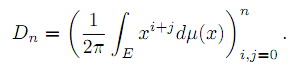In the case when E=[a,b]⊂ (-1,1) and μ satisfies the condition dμ/dx>0 a.e. on E, we investigate asymptotic behavior of singular numbers σkn,n of the Hankel matrix Dn, where kn/n→θ∈[0,1] as n→∞. Moreover, we obtain asymptotics of the Kolmogorov, Gelfand and linear k-widths, k=kn, of the unit ball An,2 of Pn∩L2(Γ) in the space L2(μ,E), where Γ={z:|z|=1} and Pn is the class of all polynomials of the degree at most n.

5: Paper Source PDF document

Paper's Title:

Applications of Von Neumann Algebras to Rigidity Problems of (2-Step) Riemannian (Nil-)Manifolds

Author(s):

DFouman Faculty of Engineering,
College of Engineering, University of Tehran,
Iran.

Department of Mathematical Sciences,
Sharif University of Technology,
Iran
E-mail: fanai@sharif.edu

Abstract:

In this paper, basic notions of von Neumann algebra and its direct analogues in the realm of groupoids and measure spaces have been considered. By recovering the action of a locally compact Lie group from a crossed product of a von Neumann algebra, other proof of one of a geometric propositions of O'Neil and an extension of it has been proposed. Also, using the advanced exploration of nilmanifolds in measure spaces and their corresponding automorphisms (Lie algebraic derivations) a different proof of an analytic theorem of Gordon and Mao has been attained. These two propositions are of the most important ones for rigidity problems of Riemannian manifolds especially 2-step nilmanifolds.

4: Paper Source PDF document

Paper's Title:

A Coincidence Theorem for Two Kakutani Maps

Author(s):

Mircea Balaj

Department of Mathematics,
Romania.

Abstract:

In this paper we prove the following theorem: Let X be a nonempty compact convex set in a locally convex Hausdorff topological vector space, D be the set of its extremal points and F,T: XX two Kakutani maps; if for each nonempty finite subset A of D and for any x ∈ coA, F (x) coA ≠ Ř, then F and T have a coincidence point. The proof of this theorem is given first in the case when X is a simplex, then when X is a polytope and finally in the general case. Several reformulations of this result are given in the last part of the paper.

4: Paper Source PDF document

Paper's Title:

Nonlinear System of Mixed Ordered Variational Inclusions Involving XOR Operation

Author(s):

Iqbal Ahmad, Abdullah and Syed Shakaib Irfan

Department of Mechanical Engineering,
College of Engineering, Qassim University
Buraidah 51452, Al-Qassim,
Saudi Arabia.

Zakir Husain Delhi College,
University of Delhi,
JLN Marg, New Delhi- 110 002,
India.
E-mail: abdullahdu@qec.edu.sa

Department of Mathematics,
Aligarh Muslim University, Aligarh,
India.
E-mail: shakaibirfan@gmail.com

Abstract:

In this work, we introduce and solve an NSMOVI frameworks system involving XOR operation with the help of a proposed iterative algorithm in real ordered positive Hilbert spaces. We discuss the existence of a solution of a considered system of inclusions involving XOR operation by applying the resolvent operator technique with XOR operation and also study the strong convergence of the sequences generated by the considered algorithm. Further, we give a numerical example in support of our considered problem which gives the grantee that all the proposed conditions of our main result are fulfilled.

3: Paper Source PDF document

Paper's Title:

On Vector Variational Inequality Problem in Terms of Bifunctions

Author(s):

C. S. Lalitha and Monika Mehta

Department of Mathematics, Rajdhani College,
University of Delhi, Raja Garden,
Delhi 110015, India
cslalitha@rediffmail.com

Department of Mathematics, Satyawati College,
University Of Delhi, Ashok Vihar,
Phase-III, Delhi 110052, India
mridul_in@yahoo.com

Abstract:

In this paper, we consider a generalized vector variational inequality problem expressed in terms of a bifunction and establish existence theorems for this problem by using the concepts of cone convexity and cone strong quasiconvexity and employing the celebrated Fan's Lemma. We also give two types of gap functions for this problem.

3: Paper Source PDF document

Paper's Title:

The Convergence of Modified Mann-Ishikawa Iterations when Applied to an Asymptotically Pseudocontractive Map

Author(s):

S. Soltuz

Departamento de Matematicas, Universidad de Los Andes, Carrera 1
No. 18A-10, Bogota,
Colombia
and
``T. Popoviciu" Institute of Numerical Analysis
Cluj-Napoca,
Romania
smsoltuz@gmail.com
URL:http://www.uniandes.edu.co/

Abstract:

We prove that under minimal conditions the modified Mann and Ishikawa iterations converge when dealing with an asymptotically pseudocontractive map. We give an affirmative answer to the open question from C.E. Chidume and H. Zegeye, Approximate fixed point sequences and convergence theorems for asymptotically pseudocontractive mappings, J. Math. Anal. Appl., 278 (2003), 354--366.

3: Paper Source PDF document

Paper's Title:

Ulam Stability of Functional Equations

Author(s):

Stefan Czerwik and Krzysztof Król

Institute of Mathematics
Silesian University of Technology
Kaszubska 23, 44-100 Gliwice,
Poland

Stefan.Czerwik@polsl.pl
Krzysztof.Krol@polsl.pl

Abstract:

In this survey paper we present some of the main results on Ulam-Hyers-Rassias stability for important functional equations.

3: Paper Source PDF document

Paper's Title:

Stability of Almost Multiplicative Functionals

Author(s):

Norio Niwa, Hirokazu Oka, Takeshi Miura and Sin-Ei Takahasi

Faculty of Engineering, Osaka Electro-Communication University,
Neyagawa 572-8530,
Japan

Faculty of Engineering, Ibaraki University,
Hitachi 316-8511,
Japan

Department of Applied Mathematics and Physics, Graduate School of Science and Engineering,
Yamagata University,
Yonezawa 992-8510
Japan

oka@mx.ibaraki.ac.jp
miura@yz.yamagata-u.ac.jp
sin-ei@emperor.yz.yamagata-u.ac.jp

Abstract:

Let δ and p be non-negative real numbers. Let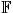be the real or complex number field and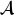a normed algebra over. If a mapping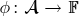satisfies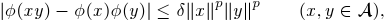then we show that φ is multiplicative or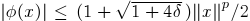for all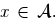If, in addition, φ satisfies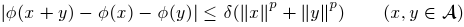for some p1, then by using Hyers-Ulam-Rassias stability of additive Cauchy equation, we show that φ is a ring homomorphism or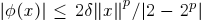for all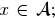In other words, φ is a ring homomorphism, or an approximately zero mapping. The results of this paper are inspired by Th.M. Rassias' stability theorem.

3: Paper Source PDF document

Paper's Title:

Real Interpolation Methods and Quasilogarithmic Operators

Author(s):

Ming Fan

School of Industrial Technology and Management,
Dalarna University, 781 88 Borlänge, Sweden

fmi@du.se
URL: http://users.du.se/~fmi

Abstract:

The purpose of this paper is to deal with nonlinear quasilogarithmic operators, which possesses the uniformly bounded commutator property on various interpolation spaces in the sense of Brudnyi-Krugljak associated with the quasi-power parameter spaces. The duality, and the domain and range spaces of these operators are under consideration. Some known inequalities for the Lebesgue integration spaces and the trace classes are carried over to the non-commutative symmetric spaces of measurable operators affiliated with a semi-finite von Neumann algebra.

3: Paper Source PDF document

Paper's Title:

Unital Compact Homomorphisms Between Extended Analytic Uniform Algebras

Author(s):

Department of Mathematics,
Faculty of Science, Arak University,
PO Box 38156-8-8349, Arak,
Iran.

m-maighany@yahoo.com

Abstract:

Let X and K be compact plane sets with KX. We denote by A(X,K) and A(X) the algebras of all continuous complex-valued functions on X which are analytic on int(K) and int(X), respectively. It is known that A(X,K) and A(X) are natural uniform algebras on X. A(X) and A(X,K) are called analytic uniform algebra and extended analytic uniform algebra on X, respectively. In this paper we study unital homomorphisms between extended analytic uniform algebras and investigate necessary and sufficient conditions for which these homomorphisms to be compact. We also determine the spectrum of unital compact endomorphisms of extended analytic uniform algebras.

3: Paper Source PDF document

Paper's Title:

Bartle Integration in Lie Algebras

Author(s):

Andreas Boukas and Philip Feinsilver

Centro Vito Volterra,
Universita di Roma Tor Vergata,
via Columbia 2, 00133 Roma,
Italy.

Department of Mathematics,
Southern Illinois University,
Carbondale, Illinois 62901,
USA.

E-mail: andreasboukas@yahoo.com
E-mail:  pfeinsil@math.siu.edu

Abstract:

Using Bartle's bilinear vector integral we define stochastic integrals of bounded operator valued functions with respect to Stieltjes measures associated with the generators of the Heisenberg and Finite Difference Lie algebras. Our definition also covers the Square of White Noise and sl/2 Lie algebras.

3: Paper Source PDF document

Paper's Title:

Ostrowski Type Inequalities for Lebesgue Integral: a Survey of Recent Results

Author(s):

Sever S. Dragomir1,2

1Mathematics, School of Engineering & Science
Victoria University, PO Box 14428
Melbourne City, MC 8001,
Australia
E-mail: sever.dragomir@vu.edu.au

2DST-NRF Centre of Excellence in the Mathematical and Statistical Sciences,
School of Computer Science & Applied Mathematics,
University of the Witwatersrand,
Private Bag 3, Johannesburg 2050,
South Africa
URL: http://rgmia.org/dragomir

Abstract:

The main aim of this survey is to present recent results concerning Ostrowski type inequalities for the Lebesgue integral of various classes of complex and real-valued functions. The survey is intended for use by both researchers in various fields of Classical and Modern Analysis and Mathematical Inequalities and their Applications, domains which have grown exponentially in the last decade, as well as by postgraduate students and scientists applying inequalities in their specific areas.

3: Paper Source PDF document

Paper's Title:

Inequalities for Discrete F-Divergence Measures: A Survey of Recent Results

Author(s):

Sever S. Dragomir1,2

1Mathematics, School of Engineering & Science
Victoria University, PO Box 14428
Melbourne City, MC 8001,
Australia
E-mail: sever.dragomir@vu.edu.au

2DST-NRF Centre of Excellence in the Mathematical and Statistical Sciences,
School of Computer Science & Applied Mathematics,
University of the Witwatersrand,
Private Bag 3, Johannesburg 2050,
South Africa
URL: http://rgmia.org/dragomir

Abstract:

In this paper we survey some recent results obtained by the author in providing various bounds for the celebrated f-divergence measure for various classes of functions f. Several techniques including inequalities of Jensen and Slater types for convex functions are employed. Bounds in terms of Kullback-Leibler Distance, Hellinger Discrimination and Varation distance are provided. Approximations of the f-divergence measure by the use of the celebrated Ostrowski and Trapezoid inequalities are obtained. More accurate approximation formulae that make use of Taylor's expansion with integral remainder are also surveyed. A comprehensive list of recent papers by several authors related this important concept in information theory is also included as an appendix to the main text.

3: Paper Source PDF document

Paper's Title:

Introducing the Dorfmanian: A Powerful Tool for the Calculus Of Variations

Author(s):

Olivier de La Grandville

Department of Management Science and Engineering,
Stanford University,
475 Via Ortega, Stanford, CA 94305,
U. S. A.

E-mail: odelagrandville@gmail.com

Abstract:

We show how a modified Hamiltonian proposed by Robert Dorfman  to give intuitive sense to the Pontryagin maximum principle can be extended to easily obtain all high-order equations of the calculus of variations. This new concept is particularly efficient to determine the differential equations leading to the extremals of functionals defined by n-uple integrals, while a traditional approach would require -- in some cases repeatedly -- an extension of Green's theorem to n-space.
Our paper is dedicated to the memory of Robert Dorfman (1916 - 2002).

3: Paper Source PDF document

Paper's Title:

Iterative Algorithm for Split Generalized Mixed Equilibrium Problem Involving Relaxed Monotone Mappings in Real Hilbert Spaces

Author(s):

1U.A. Osisiogu, F.L. Adum, and 2C. Izuchukwu

1Department of Mathematics and Computer Science,
Ebonyi State University, Abakaliki,
Nigeria.

2School of Mathematics, Statistics and Computer Science,
University of KwaZulu-Natal, Durban,
South Africa.
E-mail: izuchukwuc@ukzn.ac.za, izuchukwu_c@yahoo.com

Abstract:

The main purpose of this paper is to introduce a certain class of split generalized mixed equilibrium problem involving relaxed monotone mappings. To solve our proposed problem, we introduce an iterative algorithm and obtain its strong convergence to a solution of the split generalized mixed equilibrium problems in Hilbert spaces. As special cases of the proposed problem, we studied the proximal split feasibility problem and variational inclusion problem.

3: Paper Source PDF document

Paper's Title:

A Generalization of Ostrowski's Inequality for Functions of Bounded Variation via a Parameter

Author(s):

Seth Kermausuor

Department of Mathematics and Computer Science,
Alabama State University,
Montgomery, AL 36101,
USA.
E-mail: skermausour@alasu.edu

Abstract:

In this paper, we provide a generalization of the Ostrowski's inequality for functions of bounded variation for k points via a parameter λ∈[0,1]. As a by product, we consider some particular cases to obtained some interesting inequalities in these directions. Our results generalizes some of the results by Dragomir in [S. S. DRAGOMIR, The Ostrowski inequality for mappings of bounded variation, Bull. Austral. Math. Soc., 60 (1999), pp. 495--508.]

3: Paper Source PDF document

Paper's Title:

Extension of Factorization Theorems of Maurey to s-positively Homogeneous Operators

Author(s):

Abdelmoumen Tiaiba

Department of Physics,
University of M'sila,
Algeria.
E-mail: tiaiba05@yahoo.fr

Abstract:

In the present work, we prove that the class of s-positively homogeneous operators is a Banach space. As application, we give the generalization of some Maurey factorization theorems to T which is a s-positively homogeneous operator from X a Banach space into Lp. Where we establish necessary and sufficient conditions to proof that T factors through Lq. After this we give extend result of dual factorization theorem to same class of operators above.

2: Paper Source PDF document

Paper's Title:

Multivalued Equilibrium Problems with Trifunction

Author(s):

Etisalat College of Engineering, P.O. Box 980, Sharjah, United Arab Emirates
noor@ece.ac.ae

Abstract:

In this paper, we use the auxiliary principle technique to suggest some new classes of iterative algorithms for solving multivalued equilibrium problems with trifunction. The convergence of the proposed methods either requires partially relaxed strongly monotonicity or pseudomonotonicity. As special cases, we obtain a number of known and new results for solving various classes of equilibrium and variational inequality problems. Since multivalued equilibrium problems with trifunction include equilibrium, variational inequality and complementarity problems as specials cases, our results continue to hold for these problems.

2: Paper Source PDF document

Paper's Title:

Positive Solutions for Systems of Three-point Nonlinear Boundary Value Problems

Author(s):

J. Henderson and S. K. Ntouyas

Department of Mathematics, Baylor University
Waco, Texas
76798-7328 USA.
Johnny_Henderson@baylor.edu
URL: http://www3.baylor.edu/~Johnny_Henderson

Department of Mathematics, University of Ioannina
451 10 Ioannina,
Greece.
sntouyas@cc.uoi.gr
URL: http://www.math.uoi.gr/~sntouyas

Abstract:

Values of λ are determined for which there exist positive solutions of the system of three-point boundary value problems, u''(t)+ λa(t)f(v(t))=0, v''(t)+λb(t)g(u(t))=0, for 0 < t <1, and satisfying, u(0) = 0, u(1)=α u(η), v(0) = 0, v(1)=α v(η). A Guo-Krasnosel'skii fixed point theorem is applied.

2: Paper Source PDF document

Paper's Title:

Contact With Adhesion between a Deformable Body and a Foundation

Author(s):

B. Teniou and M. Sofonea

Laboratoire de Mathematiques Appliquées et Modélisation,
Université Mentouri, Constantine 25000,
Algeria
tenioubou2@yahoo.fr

Laboratoire de Mathématiques et Physiques pour les Systémes,
Univesité de Perpignan,
France.
sofonea@univ-perp.fr

Abstract:

The aim of this work is study a dynamic contact problem between a deformable body and a foundation where the deformations are supposed to be small. The contact is with adhesion and normal compliance. The behavior of this body is modeled by a nonlinear elastic-visco-plastic law. The evolution of bonding field is described by a nonlinear differential equation. We derive a variational formulation of the contact problem and we prove the existence and uniqueness of its solution. The proof is based on the construction of three intermediate problems and then we construct a contraction mapping whose unique fixed point will be the weak solution of the mechanical problem.

2: Paper Source PDF document

Paper's Title:

Nontrivial Solutions of Singular Superlinear Three-point Boundary Value Problems at Resonance

Author(s):

Feng Wang, Fang Zhang

School of Mathematics and Physics,
Changzou University,
Changzhou, 213164,
China.
fengwang188@163.com

Abstract:

The singular superlinear second order three-point boundary value problems at resonance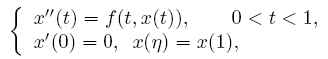are considered under some conditions concerning the first eigenvalues corresponding to the relevant linear operators, where n ∈ (0,1) is a constant, f is allowed to be singular at both t=0 and t=1. The existence results of nontrivial solutions are given by means of the topological degree theory.

2: Paper Source PDF document

Paper's Title:

Shape Diagrams for 2D Compact Sets - Part I: Analytic Convex Sets.

Author(s):

S. Rivollier, J. Debayle and J.-C. Pinoli

Ecole Nationale Supérieure des Mines de Saint-Etienne,

CIS - LPMG, UMR CNRS 5148, 158 cours Fauriel,

42023 Saint-Etienne Cedex 2, France.

Abstract:

Shape diagrams are representations in the Euclidean plane introduced to study 3-dimensional and 2-dimensional compact convex sets. Such a set is represented by a point within a shape diagram whose coordinates are morphometrical functionals defined as normalized ratios of geometrical functionals. Classically, the geometrical functionals are the area, the perimeter, the radii of the inscribed and circumscribed circles, and the minimum and maximum Feret diameters. They allow thirty-one shape diagrams to be built. Most of these shape diagrams can also been applied to more general compact sets than compact convex sets. Starting from these six classical geometrical functionals, a detailed comparative study has been performed in order to analyze the representation relevance and discrimination power of these thirty-one shape diagrams. The purpose of this paper is to present the first part of this study, by focusing on analytic compact convex sets. A set will be called analytic if its boundary is piecewise defined by explicit functions in such a way that the six geometrical functionals can be straightforwardly calculated. The second and third part of the comparative study are published in two following papers [19.20]. They are focused on analytic simply connected sets and convexity discrimination for analytic and discretized simply connected sets, respectively.

2: Paper Source PDF document

Paper's Title:

Shape Diagrams for 2D Compact Sets - Part II: Analytic Simply Connected Sets.

Author(s):

S. Rivollier, J. Debayle and J.-C. Pinoli

Ecole Nationale Supérieure des Mines de Saint-Etienne,

CIS - LPMG, UMR CNRS 5148, 158 cours Fauriel,

42023 Saint-Etienne Cedex 2, France.

Abstract:

Shape diagrams are representations in the Euclidean plane introduced to study 3-dimensional and 2-dimensional compact convex sets. However, they can also been applied to more general compact sets than compact convex sets. A compact set is represented by a point within a shape diagram whose coordinates are morphometrical functionals defined as normalized ratios of geometrical functionals. Classically, the geometrical functionals are the area, the perimeter, the radii of the inscribed and circumscribed circles, and the minimum and maximum Feret diameters. They allow twenty-two shape diagrams to be built. Starting from these six classical geometrical functionals, a detailed comparative study has been performed in order to analyze the representation relevance and discrimination power of these twenty-two shape diagrams. The first part of this study is published in a previous paper 16. It focused on analytic compact convex sets. A set will be called analytic if its boundary is piecewise defined by explicit functions in such a way that the six geometrical functionals can be straightforwardly calculated. The purpose of this paper is to present the second part, by focusing on analytic simply connected compact sets. The third part of the comparative study is published in a following paper 17. It is focused on convexity discrimination for analytic and discretized simply connected compact sets.

2: Paper Source PDF document

Paper's Title:

Shape Diagrams for 2D Compact Sets - Part III: Convexity Discrimination for Analytic and Discretized Simply Connected Sets.

Author(s):

S. Rivollier, J. Debayle and J.-C. Pinoli

Ecole Nationale Supérieure des Mines de Saint-Etienne,
CIS - LPMG, UMR CNRS 5148, 158 cours Fauriel,
42023 Saint-Etienne Cedex 2, France.

Abstract:

Shape diagrams are representations in the Euclidean plane introduced to study 3-dimensional and 2-dimensional compact convex sets. However, they can also been applied to more general compact sets than compact convex sets. A compact set is represented by a point within a shape diagram whose coordinates are morphometrical functionals defined as normalized ratios of geometrical functionals. Classically, the geometrical functionals are the area, the perimeter, the radii of the inscribed and circumscribed circles, and the minimum and maximum Feret diameters. They allow twenty-two shape diagrams to be built. Starting from these six classical geometrical functionals, a detailed comparative study has been performed in order to analyze the representation relevance and discrimination power of these twenty-two shape diagrams. The two first parts of this study are published in previous papers 8,9. They focus on analytic compact convex sets and analytic simply connected compact sets, respectively. The purpose of this paper is to present the third part, by focusing on the convexity discrimination for analytic and discretized simply connected compact sets..

2: Paper Source PDF document

Paper's Title:

A One-Line Derivation of the Euler and Ostrogradski Equations

Author(s):

Olivier de La Grandville

Stanford University,
Department of Management Science and Engineering,
Stanford, CA 94305,
U. S. A

Abstract:

At the very heart of major results of classical physics, the Euler and Ostrogradski equations have apparently no intuitive interpretation. In this paper we show that this is not so. Relying on Euler's initial geometric approach, we show that they can be obtained through a direct reasoning that does not imply any calculation. The intuitive approach we suggest offers two benefits: it gives immediate significance to these fundamental second-order non-linear differential equations; and second, it allows to obtain a property of the calculus of variations that does not seem to have been uncovered until now: the Euler and Ostrogradski equations can be derived not necessarily by giving a variation to the optimal function -- as is always done; one could equally well start by giving a variation to their derivative(s).

2: Paper Source PDF document

Paper's Title:

On Eigenvalues and Boundary Curvature of the C*-algebra Numerical Rang

Author(s):

M. T. Heydari

Department of Mathematics,
College of Sciences,
Yasouj University,
Yasouj, 75914-74831,
Iran.

E-mail: heydari@yu.ac.ir

Abstract:

Let A be a C*-algebra with unit 1 and a∈A be a nilpotent. By Donoghue's Theorem, all corner points of its numerical range V(a) belong to the spectrum σ(a). It is therefore natural to expect that, more generally, the distance from a point p on the boundary ∂ V(a) of V(a) to σ(a) should be in some sense bounded by the radius of curvature of ∂ V(a) at p.

2: Paper Source PDF document

Paper's Title:

Generalized Weighted Trapezoid and Grüss Type Inequalities on Time Scales

Author(s):

Eze Raymond Nwaeze

Department of Mathematics,
Tuskegee University,
Tuskegee, AL 36088,
USA.
E-mail: enwaeze@mytu.tuskegee.edu

Abstract:

In this work, we obtain some new generalized weighted trapezoid and Grüss type inequalities on time scales for parameter functions. Our results give a broader generalization of the results due to Pachpatte in . In addition, the continuous and discrete cases are also considered from which, other results are obtained.

2: Paper Source PDF document

Paper's Title:

The Concept of Convergence for 2-Dimensional Subspaces Sequence in Normed Spaces

Author(s):

M. Manuharawati, D. N. Yunianti, M. Jakfar

Mathematics Department, Universitas Negeri Surabaya,
Jalan Ketintang Gedung C8,
Surabaya 60321,
Indonesia.
E-mail: manuharawati@unesa.ac.id, dwiyunianti@unesa.ac.id,

Abstract:

In this paper, we present a concept of convergence of sequence, especially, of 2-dimensional subspaces of normed spaces. The properties of the concept are established. As consequences of our definition in an inner product space, we also obtain the continuity property of the angle between two 2-dimensional subspaces of inner product spaces.

1: Paper Source PDF document

Paper's Title:

Long Time Behavior for a Viscoelastic Problem with a Positive Definite Kernel

Author(s):

Nasser-eddine Tatar

King Fahd University of Petroleum and Mineral, Department of Mathematical Sciences,
Dhahran, 31261 Saudi Arabia

Abstract:

We study the asymptotic behavior of solutions for an integro-differential problem which arises in the theory of viscoelasticity. It is proved that solutions go to rest in an exponential manner under new assumptions on the relaxation function in the memory term. In particular, we consider a new family of kernels which are not necessarily decreasing.

1: Paper Source PDF document

Paper's Title:

Essential Random Fixed Point Set of Random Operators

Author(s):

Ismat Beg

Centre for Advanced Studies in Mathematics,
Lahore University of Management Sciences (LUMS),
54792-Lahore, PAKISTAN. ibeg@lums.edu.pk
URL: http://web.lums.edu.pk/~ibeg

Abstract:

We obtain necessary and sufficient conditions for the existence of essential random fixed point of a random operator defined on a compact metric space. The structure of the set of essential random fixed points is also studied.

1: Paper Source PDF document

Paper's Title:

Positive Periodic Time-Scale Solutions for Functional Dynamic Equations

Author(s):

Douglas R. Anderson and Joan Hoffacker

Department of Mathematics and Computer Science
Concordia College
andersod@cord.edu
URL:
http://www.cord.edu/faculty/andersod/

Department of Mathematical Sciences
Clemson University
Clemson, SC 29634 USA
johoff@clemson.edu
URL:
http://www.math.clemson.edu/facstaff/johoff.htm

Abstract:

Using Krasnoselskii's fixed point theorem, we establish the existence of positive periodic solutions to two pairs of related nonautonomous functional delta dynamic equations on periodic time scales, and then extend the discussion to higher-dimensional equations. Two pairs of corresponding nabla equations are also provided in an analogous manner.

1: Paper Source PDF document

Paper's Title:

Normalized Truncated Levy models applied to the study of Financial Markets

Author(s):

M. C. Mariani, K. Martin, D. W. Dombrowski and D. Martinez

Department of Mathematical Sciences and Department of Finance,
New Mexico State University, P.O. Box 30001
Department 3MB Las Cruces, New Mexico 88003-8001
USA.
mmariani@nmsu.edu
kjmartin@nmsu.edu

Abstract:

This work is devoted to the study of the statistical properties of financial instruments from developed markets. We performed a new analysis of the behavior of companies corresponding to the DJIA index, and of the index itself, by using a normalized Truncated Levy walk model. We conclude that the Truncated Levy distribution describes perfectly the evolution of the companies and of the index near a crash.

1: Paper Source PDF document

Paper's Title:

Existence Results for Perturbed Fractional Differential Inclusions

Author(s):

Y.-K. Chang

Department of Mathematics,
Lanzhou Jiaotong University, Lanzhou, Gansu 730070, People's
Republic of China
lzchangyk@163.com

Abstract:

This paper is mainly concerned with the following fractional differential inclusions with boundary condition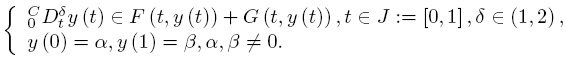A sufficient condition is established for the existence of solutions of the above problem by using a fixed point theorem for multivalued maps due to Dhage. Our result is proved under the mixed generalized Lipschitz and Carathéodory conditions.

1: Paper Source PDF document

Paper's Title:

Regular Variation on Time Scales and Dynamic Equations

Author(s):

Pavel Řehák

Institute of Mathematics, Academy of Sciences of the Czech Republic
Žižkova 22, CZ61662 Brno,
Czech Republic
rehak@math.muni.cz
URL:http://www.math.muni.cz/~rehak

Abstract:

The purpose of this paper is twofold. First, we want to initiate a study of regular variation on time scales by introducing this concept in such a way that it unifies and extends well studied continuous and discrete cases. Some basic properties of regularly varying functions on time scales will be established as well. Second, we give conditions under which certain solutions of linear second order dynamic equations are regularly varying. Open problems and possible directions for a future research are discussed, too.

1: Paper Source PDF document

Paper's Title:

Inequalities for the Čebyšev Functional of Two Functions of Selfadjoint Operators in Hilbert Spaces

Author(s):

S. S. Dragomir

School of Engineering and Science
Victoria University, PO 14428
Melbourne City MC, Victoria 8001,
Australia

sever.dragomir@vu.edu.au
URL
: http://www.staff.vu.edu.au/RGMIA/dragomir/

Abstract:

Some recent inequalities for the Čebyšev functional of two functions of selfadjoint linear operators in Hilbert spaces, under suitable assumptions for the involved functions and operators, are surveyed.

1: Paper Source PDF document

Paper's Title:

Hyperbolic Barycentric Coordinates

Author(s):

Abraham A. Ungar

Department of Mathematics, North Dakota State University,
Fargo, ND 58105,
USA
Abraham.Ungar@ndsu.edu
URL
: http://math.ndsu.nodak.edu/faculty/ungar/

Abstract:

A powerful and novel way to study Einstein's special theory of relativity and its underlying geometry, the hyperbolic geometry of Bolyai and Lobachevsky, by analogies with classical mechanics and its underlying Euclidean geometry is demonstrated. The demonstration sets the stage for the extension of the notion of barycentric coordinates in Euclidean geometry, first conceived by Möbius in 1827, into hyperbolic geometry. As an example for the application of hyperbolic barycentric coordinates, the hyperbolic midpoint of any hyperbolic segment, and the centroid and orthocenter of any hyperbolic triangle are determined.

1: Paper Source PDF document

Paper's Title:

Positive Solutions to a System of Boundary Value Problems for Higher-Dimensional Dynamic Equations on Time Scales

Author(s):

I. Y. Karaca

Department of Mathematics,
Ege University,
35100 Bornova, Izmir,
Turkey

ilkay.karaca@ege.edu.tr

URL: http://ege.edu.tr

Abstract:

In this paper, we consider the system of boundary value problems for higher-dimensional dynamic equations on time scales. We establish criteria for the existence of at least one or two positive solutions. We shall also obtain criteria which lead to nonexistence of positive solutions. Examples applying our results are also given.

1: Paper Source PDF document

Paper's Title:

Some Operator Order Inequalities for Continuous Functions of Selfadjoint Operators in Hilbert Spaces

Author(s):

S. S. Dragomir1,2 and Charles E. M. Pearce3

1Mathematics, School of Engineering & Science,
Victoria University,
PO Box 14428, Melbourne City, MC 8001,
Australia.

2School of Computational & Applied Mathematics,
University of the Witwatersrand,
Private Bag 3, Johannesburg 2050,
South Africa.

sever.dragomir@vu.edu.au
URL: http://rgmia.org/dragomir

3School of Mathematical Sciences,
Australia

Abstract:

Various bounds in the operator order for the following operator transform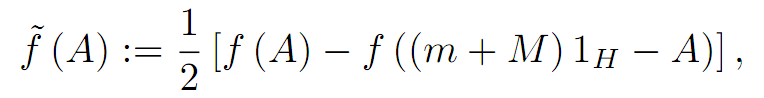where A is a selfadjoint operator in the Hilbert space H with the spectrum
Sp( A) ⊆ [ m,M] and f:[m,M] ->  C is a continuous function on [m,M]  are given. Applications for the power and logarithmic functions are provided as well.

1: Paper Source PDF document

Paper's Title:

End-Point and Transversality Conditions in the Calculus of Variations: Derivations through Direct Reasoning

Author(s):

Olivier de La Grandville

Stanford University,
Department of Management Science and Engineering,
475 Via Ortega, Stanford, CA 94305,
U. S. A.

E-mail: ola@stanford.edu

Abstract:

We offer an intuitive explanation of the end-point and transversality conditions that complement the Euler equation in the calculus of variations. Our reasoning is based upon the fact that any variation given to an optimal function must entail a zero net gain to the functional, all consequences of implied changes in its derivative being fully taken into account.

1: Paper Source PDF document

Paper's Title:

Some New Generalizations of Jensen's Inequality with Related Results and Applications

Author(s):

Steven G. From

Department of Mathematics

E-mail: sfrom@unomaha.edu

Abstract:

In this paper, some new generalizations of Jensen's inequality are presented. In particular, upper and lower bounds for the Jensen gap are given and compared analytically and numerically to previously published bounds for both the discrete and continuous Jensen's inequality cases. The new bounds compare favorably to previously proposed bounds. A new method based on a series of locally linear interpolations is given and is the basis for most of the bounds given in this paper. The wide applicability of this method will be demonstrated. As by-products of this method, we shall obtain some new Hermite-Hadamard inequalities for functions which are 3-convex or 3-concave. The new method works to obtain bounds for the Jensen gap for non-convex functions as well, provided one or two derivatives of the nonlinear function are continuous. The mean residual life function of applied probability and reliability theory plays a prominent role in construction of bounds for the Jensen gap. We also present an exact integral representation for the Jensen gap in the continuous case. We briefly discuss some inequalities for other types of convexity, such as convexity in the geometric mean, and briefly discuss applications to reliability theory.

1: Paper Source PDF document

Paper's Title:

On operators for which T2≥-T*2

Author(s):

1Department of Mathematics,
University of El Oued 39000,
Algeria
E-mail: guesbamessaoud2@gmail.com

2Department of Mathematics,
University of Msila 28000,
Algeria

Abstract:

In this paper we introduce the new class of operators for which T2 -T*2 acting on a complex Hilbert space H. We give some basic properties of these operators. we study the relation between the class and some other well known classes of operators acting on H.

1: Paper Source PDF document

Paper's Title:

Euler Series Solutions for Linear Integral Equations

Author(s):

Department of Mathematics,
University of Msila 28000,
ALGERIA.
E-mail: dilmiistapha@yahoo.fr

Abstract:

In this work, we seek the approximate solution of linear integral equations by truncation Euler series approximation. After substituting the Euler expansions for the given functions of the equation and the unknown one, the equation reduces to a linear system, the solution of this latter gives the Euler coefficients and thereafter the solution of the equation. The convergence and the error analysis of this method are discussed. Finally, we compare our numerical results by others.

1: Paper Source PDF document

Paper's Title:

MSplit Equality for Monotone Inclusion Problem and Fixed Point Problem in Real Banach Spaces

Author(s):

1,2Christian Chibueze Okeke, 3Abdumalik Usman Bello, 1Chinedu Izuchukwu, and 1Oluwatosin Temitope Mewomo

1School of Mathematics,
Statistics and Computer Science,
University of KwaZulu-Natal, Durban,
South Africa.
E-mail: okekec@ukzn.ac.za
E-mail: izuchukwuc@ukzn.ac.za
E-mail: mewomoo@ukzn.ac.za

2DST-NRF Center of Excellence in Mathematical and Statistical Sciences (CoE-Mass)
Johannesburg,
South Africa.

3Federal University,
Dutsin-Ma, Katsina State,
Nigeria.
E-mail: uabdulmalik@fudutsinma.edu.ng

Abstract:

In this paper a new iterative algorithm for approximating a common solution of split equality monotone inclusion problem and split equality fixed point problem is introduced. Using our algorithm, we state and prove a strong convergence theorem for approximating an element in the intersection of the set of solutions of a split equality monotone inclusion problem and the set of solutions of a split equality fixed point problem for right Bregman strongly nonexpansive mappings in the setting of p-uniformly convex Banach spaces which are also uniformly smooth. We also give some applications.

1: Paper Source PDF document

Paper's Title:

Author(s):

Department of Mathematics,
University of Msila 28000,
ALGERIA.
E-mail: smatilotfi@gmail.com

Abstract:

In this paper, we present some sufficient conditions which ensure the compactness, the normality, the positivity, the closedness and the skew self-adjointness of the unbounded Nadir's operator on a Hilbert space. We get also when the measurement of its adjointness is null and other related results are also established.

1: Paper Source PDF document

Paper's Title:

Numerical Radius Isometries between Hermitian Banach Algebras

Author(s):

Mohamed Mabrouk and Ashwaq Albideewi

Department of Mathematics,
University of Gabes,
6072 Zrig, Gabes,
Tunisia.
E-mail: mohamed.mabrouk@fsg.rnu.tn

Department of Mathematics,
College of Applied Sciences,
Umm Al-Qura University,
P. O. Box 715,
Makkah 21955,
Saudi Arabia.
Email: Ashwaq.F.B@hotmail.com

Abstract:

In the case of C*-algebras, the author in  showed that any linear unital and surjective numerical radius isometry is a Jordan *-isomorphism. In this paper, we generalize this result to the case of Hermitian Banach algebras.

1: Paper Source PDF document

Paper's Title:

The Influence of Fluid Pressure in Macromechanical Cochlear Model

Author(s):

F. E. Aboulkhouatem1, F. Kouilily1, N. Achtaich1, N. Yousfi1 and M. El Khasmi2

1Department of Mathematics and Computer Science, Faculty of Sciences
Ben M'sik, Hassan II University, Casablanca,
Morocco.

2Department of Biology, Faculty of Sciences
Ben M'sik, Hassan II University, Casablanca,
Morocco.

E-mail: fatiaboulkhouatem@gemail.com
URL: http://www.fsb.univh2c.ma/

Abstract:

An increase of pressure in the structure of cochlea may cause a hearing loss. In this paper, we established the relationship between the fluid pressure and the amplitude of displacement of Basilar Membrane to clarify the mechanisms of hearing loss caused by increasing of this pressure. So, a mathematical cochlear model was formulated using finite difference method in order to explain and demonstrate this malfunction in passive model. Numerical simulations may be considered as helpful tools which may extend and complete the understanding of a cochlea dysfunction.

1: Paper Source PDF document

Paper's Title:

The Jacobson Density Theorem for Non-Commutative Ordered Banach Algebras

Author(s):

Kelvin Muzundu

University of Zambia,
Deparment of Mathematics and Statistics,
P.O. Box 32379, Lusaka,
Zambia.
E-mail: kmzundu@gmail.com

Abstract:

The Jacobson density theorem for general non-commutative Banach algebras states as follows: Let π be a continuous, irreducible representation of a non-commutative Banach algebra A on a Banach space X. If x1,x2,...,xn are linearly independent in X and if y1,y2,...,yn are in X, then there exists an a A such that π(a)xi=yi for i=1,2,...,n. By considering ordered Banach algebras A and ordered Banach spaces X, we shall establish an order-theoretic version of the Jacobson density theorem.

1: Paper Source PDF document

Paper's Title:

Several Applications of a Local Non-convex Young-type Inequality

Author(s):

Loredana Ciurdariu, Sorin Lugojan

Department of Mathematics,
"Politehnica" University of Timisoara,
P-ta. Victoriei, No.2, 300006-Timisoara,
Romania.

E-mail: ltirtirau87@yahoo.com

Abstract:

A local version of the Young inequality for positive numbers is used in order to deduce some inequalities about determinants and norms for real quadratic matrices and norms of positive operators on complex Hilbert spaces.

1: Paper Source PDF document

Paper's Title:

Author(s):

F. U. Ogbuisi

School of Mathematics, Statistics and Computer Science,
University of KwaZulu-Natal, Durban,
South Africa.

Department of Mathematics,
University of Nigeria, Nsukka,
Nigeria.
E-mail: ferdinard.ogbuisi@unn.edu.ng fudochukwu@yahoo.com

Abstract:

In this paper, we propose and analyze a type of subgradient extragradient algorithm for the approximation of a solution of variational inequality problem which is also a common fixed point of an infinite family of relatively nonexpansive mappings in 2-uniformly convex Banach spaces which are uniformly smooth. By using the generalized projection operator, we prove a strong convergence theorem which does not require the prior knowledge of the Lipschitz constant of cost operator. We further applied our result to constrained convex minimization problem, convex feasibility problem and infinite family of equilibrium problems. Our results improve and complement related results in 2-uniformly convex and uniformly smooth Banach spaces and Hilbert spaces.

1: Paper Source PDF document

Paper's Title:

Construction of a Frame Multiresolution Analysis on Locally Compact Abelian Groups

Author(s):

R. Kumar and Satyapriya

Department of Mathematics,
Kirori Mal College,
University of Delhi,
Delhi,
India.
E-mail: rajkmc@gmail.com

Department of Mathematics,
University of Delhi,
Delhi,
India.
E-mail: kmc.satyapriya@gmail.com

Abstract:

The frame multiresolution analysis (FMRA) on locally compact Abelian groups has been studied and the results concerning classical MRA have been worked upon to obtain new results. All the necessary conditions, which need to be imposed on the scaling function φ to construct a wavelet frame via FMRA, have been summed up. This process of construction of FMRA has aptly been illustrated by sufficient examples.

1: Paper Source PDF document

Paper's Title:

Coexisting Attractors and Bubbling Route to Chaos in Modified Coupled Duffing Oscillators

Author(s):

B. Deruni1, A. S. Hacinliyan1,2, E. Kandiran3, A. C. Keles2, S. Kaouache4, M.-S. Abdelouahab4, N.-E. Hamri4

1Department of Physics,
University of Yeditepe,
Turkey.

2Department of Information Systems and Technologies,
University of Yeditepe,
Turkey

3Department of Software Development,
University of Yeditepe,
Turkey.

4Laboratory of Mathematics and their interactions,
University Center of Abdelhafid Boussouf,
Mila 43000,
Algeria.

E-mail: berc890@gmail.com
ahacinliyan@yeditepe.edu.tr
engin.kandiran@yeditepe.edu.tr
cihan.keles@yeditepe.edu.tr
s.kaouache@centr-univ-mila.dz
medsalah3@yahoo.fr
n.hamri@centre-univ-mila.dz

Abstract:

In this article dynamical behavior of coupled Duffing oscillators is analyzed under a small modification. The oscillators have cubic damping instead of linear one. Although single duffing oscillator has complex dynamics, coupled duffing systems possess a much more complex structure. The dynamical behavior of the system is investigated both numerically and analytically. Numerical results indicate that the system has double scroll attractor with suitable parameter values. On the other hand, bifurcation diagrams illustrate rich behavior of the system, and it is seen that, system enters into chaos with different routes. Beside classical bifurcations, bubbling route to chaos is observed for suitable parameter settings. On the other hand, Multistability of the system is indicated with the coexisting attractors, such that under same parameter setting the system shows different periodic and chaotic attractors. Moreover, chaotic synchronization of coupled oscillators is illustrated in final section.

Search and serve lasted 1 second(s).